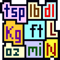iUnit Measurement Conversion
See on store
Category
Store
Last Updated
2022, Jun 07
Visibility Score
0

Jan, 2023
Worldwide

Jan, 2023
Worldwide

## Description

iUnit is a powerful yet easy to use unit conversion app. You can convert between 1650 different units in 57 categories quickly and easily. iUnit converts between the following types of units: Acceleration Acoustic impedance Angle Angular Acceleration Area Basis Weight Capacitance Charge (Electrical) Computer Storage Concentration Conductance (Electrical) Corrosion Rate Data Transfer Rate Density Diffusitivity Distance Electrical Conductance Energy Enthalpy - mass basis Enthalpy - mol basis Enthalpy - volume basis Entropy Frequency Force Heat Capacity Heat Flux Heat Rate Heat Transfer Coefficient Liquid Solution Concentration Luminous Luminence Luminous Intensity Magnetic Flux Magnetic Flux Density Magnetic Field Strength Mass Mass Flow Rate Molar Concentration Mole Flow Rate Moment-Torque Momentum Power Pressure Radiation Activity Stress Surface Tension Temperature Thermal Conductivity Thermal expansion - linear Thermal expansion - volume Time Type (printer's) Velocity Viscosity (Kinematic) Viscosity (Dynamic) Viscosity (Oil and Water) Volume Volume Flow Rate